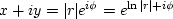Next: The exponential of a Up: Spectrum and phase Previous: Instantaneous frequency

# SPECTRAL FACTORIZATION

The spectral factorization" problem arises in a variety of physical contexts. It is this: given a spectrum, find a minimum-phase wavelet that has that spectrum. We will see how to make this wavelet, and we will recognize that it is unique. (It is unique except for a trivial aspect. The negative of any wavelet has the same spectrum as the wavelet, and, more generally, any wavelet can be multiplied by any complex number of unit magnitude, such as, etc.)

First consider the simpler problem in which the wavelet need not be causal. We can easily find a symmetric wavelet with any spectrum (which by definition is an energy or power). We simply take the square root of the spectrum--this is the amplitude spectrum. We then inverse transform the amplitude spectrum to the time domain, and we have a symmetric wavelet with the desired spectrum.

The prediction-error filter discussed in chapteris theoretically obtainable by spectral factorization of an inverse spectrum. The Kolmogoroff method of spectral factorization, which we will be looking at here, is much faster than the time-domain, least-squares methods considered in chapterand the least-squares methods given in FGDP. Its speed motivates its widespread practical use.mpsamples
Figure 8
Left are given wavelets, and right are minimum-phase equivalents.

Some simple examples of spectral factorization are given in Figure 8. For all but the fourth signal, the spectrum of the minimum-phase wavelet clearly matches that of the input. Wavelets are shifted to t=0 and turned backwards. In the fourth case, the waveshape changes into a big pulse at zero lag. As the Robinson theorem introduced on pagesuggests, minimum-phase wavelets tend to decay rapidly after a strong onset. I imagined that hand-drawn wavelets with a strong onset would rarely turn out to be perfectly minimum-phase, but when I tried it, I was surprised at how easy it seemed to be to draw a minimum-phase wavelet. This is shown on the bottom of Figure 8.

To begin understanding spectral factorization, notice that the polar form of any complex number puts the phase into the exponential, i.e.,.So we look first into the behavior of exponentials and logarithms of Fourier transforms.Next: The exponential of a Up: Spectrum and phase Previous: Instantaneous frequency
Stanford Exploration Project
10/21/1998